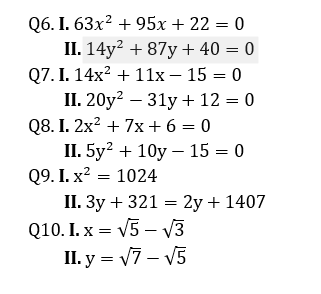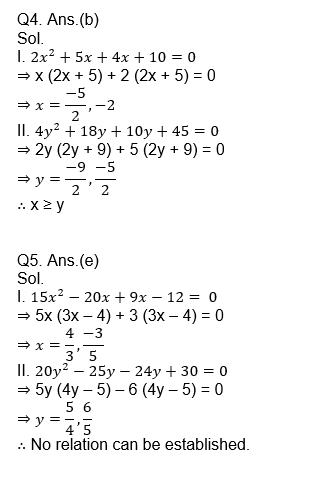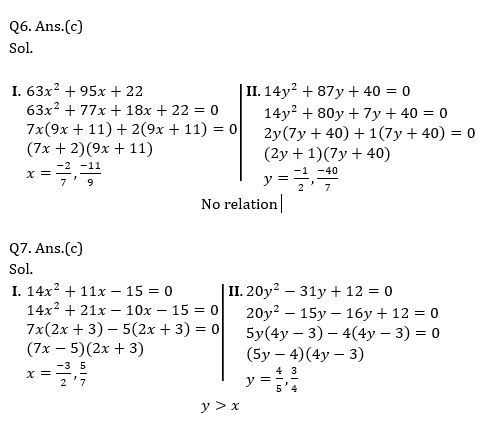# Quant Quiz(Inequality) for SBI PO PRE 2019 | 24th January 2019

Quant Quiz to improve your Quantitative Aptitude for SBI exam Reasoning, IBPS PO Reasoning , IBPS Clerk Reasoning , IBPS RRB Reasoning , , and other competitive exams.

Direction (Q1-Q10): Two equations (I) and (II) are given in each question. On the basis of these equations you have to decide the relation between ‘x’ and ‘y’ and give answer.

(a)  if x > y

(b)  if x  y

(c)  if x < y

(d)  if x y

(e)  if x = y or relation cannot be established.# Solutions## WhatsApp Group Join here

Mail us at : ambitiousbaba1@gmail.com